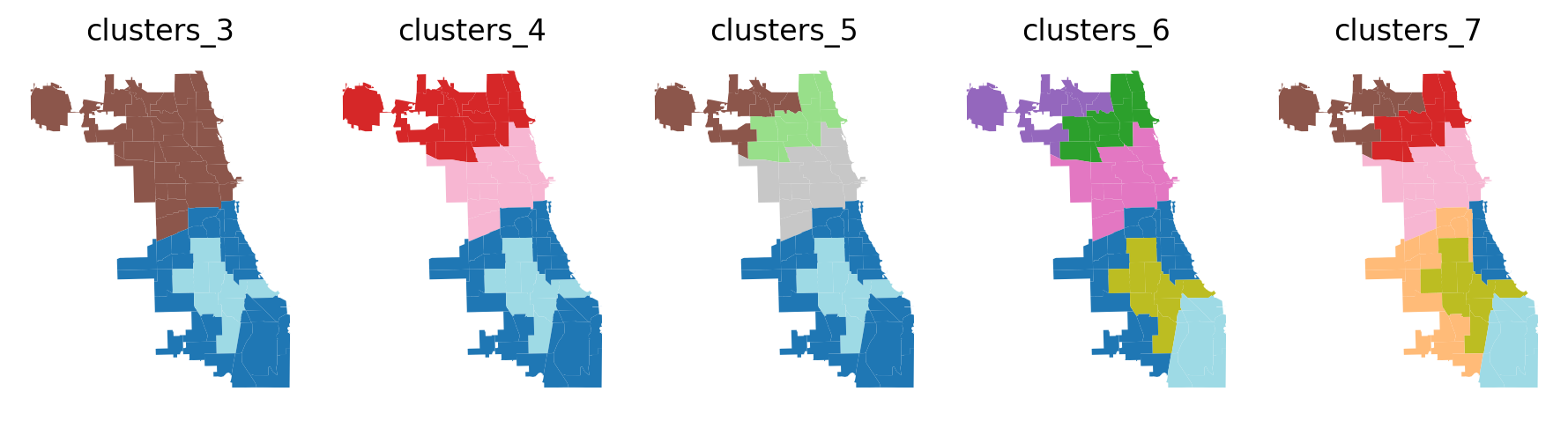# SKATER¶

## Spatial ’K’luster Analysis by Tree Edge Removal: Clustering Airbnb Spots in Chicago¶

Authors: Xin Feng, James Gaboardi

The tutorial is broken out as follows:

1. An explanation of the SKATER algorithm

2. Supporting data: Airbnb Spots in Chicago

3. Understanding parameters

4. Regionalization

5. Exploring parameters

:

%config InlineBackend.figure_format = "retina"
%watermark

Last updated: 2023-01-09T12:25:01.746631-05:00

Python implementation: CPython
Python version       : 3.10.8
IPython version      : 8.7.0

Compiler    : Clang 14.0.6
OS          : Darwin
Release     : 22.2.0
Machine     : x86_64
Processor   : i386
CPU cores   : 8
Architecture: 64bit


:

import geopandas
import libpysal
import matplotlib.pyplot as plt
import numpy
import pandas
import shapely
from sklearn.metrics import pairwise as skm
import spopt
import warnings

%matplotlib inline
%watermark -w
%watermark -iv

Watermark: 2.3.1

spopt     : 0.4.1+200.g336f235.dirty
libpysal  : 4.7.0
json      : 2.0.9
geopandas : 0.12.2
pandas    : 1.5.2
matplotlib: 3.6.2
sklearn   : 1.2.0
shapely   : 2.0.0
numpy     : 1.23.5



## 1. An explanation of the SKATER algorithm¶

SKATER (Assunção et al. 2006) is a constrained spatial regionalization algorithm based on spanning tree pruning. The number of edges is pre-specified to be cut in a continuous tree to group spatial units into contiguous regions.

The first step of SKATER is to create a connectivity graph that captures the neighbourhood relationship between the spatial objects. The cost of each edge in the graph is inversely proportional to the similarity between the regions it joins. The neighbourhood is structured by a minimum spanning tree (MST), which is a connected tree with no circuits. The next step is to partition the MST by successive removal of edges that link dissimilar regions. The final result is the division of the spatial objects into connected regions that have maximum internal homogeneity.

• RM Assunção, MC Neves, G Câmara, and C da Costa Freitas. Efficient regionalization techniques for socio-economic geographical units using minimum spanning trees. International Journal of Geographical Information Science, 20(7):797–811, 2006. doi: 10.1080/13658810600665111.

See also Dmitry Shkolnik’s tutorial of the SKATER algorithm implemented in Roger Bivand’s R package, spdep <https://r-spatial.github.io/spdep/index.html>__.

## 2. Supporting data: Airbnb Spots in Chicago¶

To illustrate Skater we utilize data on Airbnb spots in Chicago, which can be downloaded from libpysal.examples. The data is broken down into 77 communites in Chicago, Illinois from 2015, where a number of attributes are listed for each community.

:

libpysal.examples.load_example("AirBnB")

:

<libpysal.examples.base.Example at 0x166d97e50>

:

chicago = geopandas.read_file(libpysal.examples.get_path("airbnb_Chicago 2015.shp"))
chicago.shape

:

(77, 21)

:

chicago.loc

:

community                                               DOUGLAS
shape_area                                        46004621.1581
shape_len                                         31027.0545098
AREAID                                                       35
response_r                                            98.771429
accept_r                                              94.514286
rev_rating                                            87.777778
price_pp                                              78.157895
room_type                                              1.789474
num_spots                                                    38
poverty                                                    29.6
crowded                                                     1.8
dependency                                                 30.7
without_hs                                                 14.3
unemployed                                                 18.2
income_pc                                                 23791
harship_in                                                   47
num_crimes                                                 5013
num_theft                                                  1241
population                                                18238
geometry      POLYGON ((-87.60914087617012 41.84469250346108...
Name: 0, dtype: object


We can visualize the data by plotting the number of Airbnb spots in each community in the sample, using a quintile classification:

:

chicago.plot(
figsize=(7, 14),
column="num_spots",
scheme="Quantiles",
cmap="GnBu",
edgecolor="grey",
legend=True
).axis("off");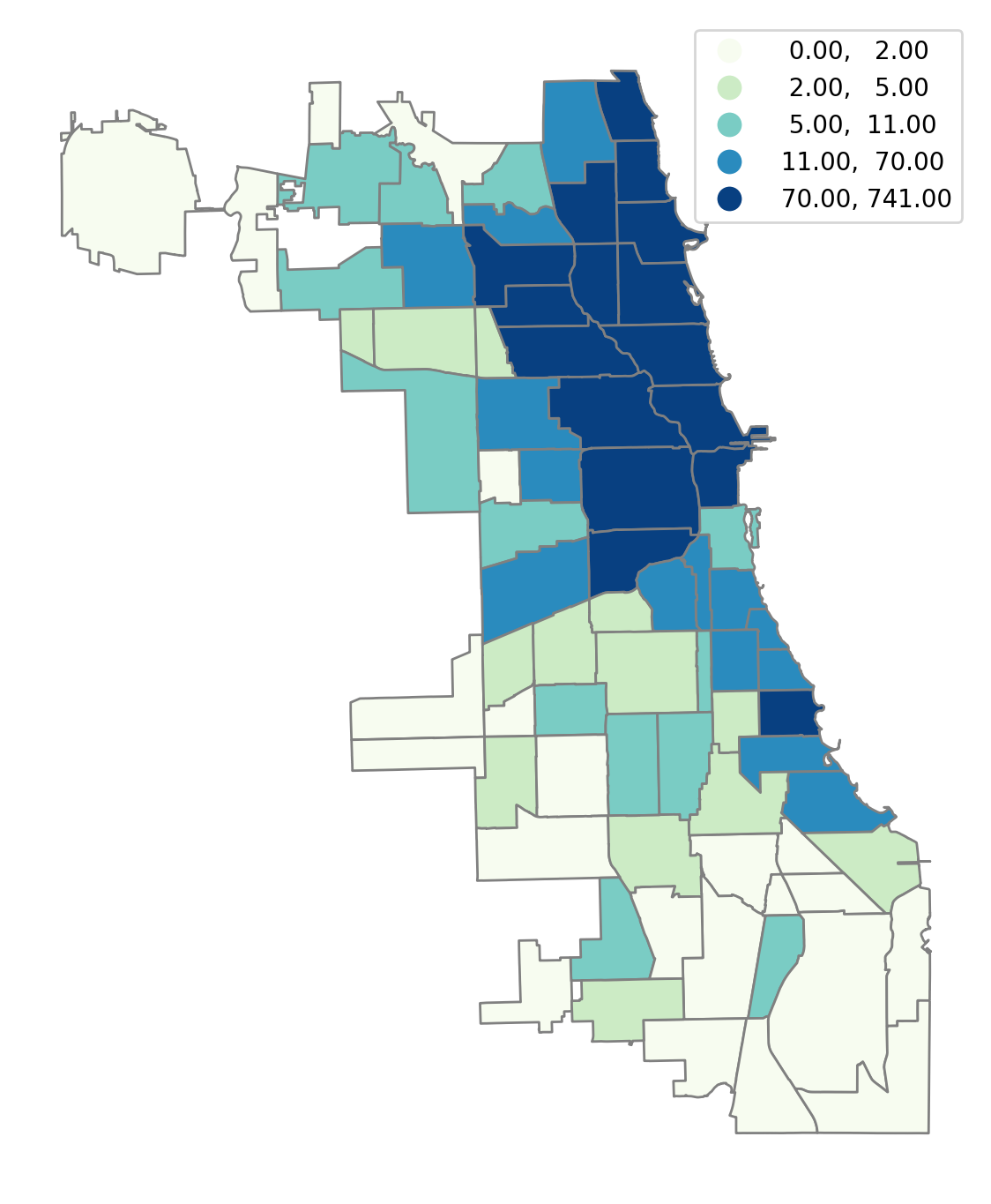## 3. Understanding parameters¶

With Skater, we can cluster these 77 communities into 5 regions such that each region consists of at least 5 communities. The homogeneity of the number of Airbnb spots per community within the regions is maximized.

We first define the variable that will be used to measure regional homogeneity, which is the number of Airbnb spots in this case.

:

attrs_name = ["num_spots"]


Next, we specify a number of other parameters that will serve as input to the skater model, including the spatial weights (to describe the relationship between the spatial objects), the number of regions to include in the solution, the minimal threshold of spatial objects in each region, etc.

A spatial weights object describes the spatial connectivity of the spatial objects:

:

w = libpysal.weights.Queen.from_dataframe(chicago)


The number of contiguous regions that we would like to group spatial units into:

:

n_clusters = 5


The minimum number of spatial objects in each region：

:

floor = 5


trace is a bool denoting whether to store intermediate labelings as the tree gets pruned.

:

trace = False


The islands keyword argument describes what is to be done with islands. It can be set to either 'ignore', which will treat each island as its own region when solving for n_clusters regions, or 'increase', which will consider each island as its own region and add to n_clusters regions.

:

islands = "increase"


We can also specify some keywords as input to the spanning forest algorithm, including:

• dissimilarity

• affinity

• A callable affinity metric between 0 and 1, which is inverted to provide a dissimilarity metric. No metric is provided as a default (None). If affinity is desired, dissimilarity must explicitly be set to None.

• reduction

• center

• verbose

• A flag for how much output to provide to the user in terms of print statements and progress bars. Set to 1 for minimal output and 2 for full output. The default is False, which provides no output.

See spopt.region.skater.SpanningForest for documentation.

:

spanning_forest_kwds = dict(
dissimilarity=skm.manhattan_distances,
affinity=None,
reduction=numpy.sum,
center=numpy.mean,
verbose=2
)


## 4. Regionalization¶

The model can then be instantiated and solved:

:

model = spopt.region.Skater(
chicago,
w,
attrs_name,
n_clusters=n_clusters,
floor=floor,
trace=trace,
islands=islands,
spanning_forest_kwds=spanning_forest_kwds
)
model.solve()

Computing Affinity Kernel took 0.00s
Computing initial MST took 0.00s
Computing connected components took 0.00s.

making cut deletion(in_node=45, out_node=67, score=3445.5)...

making cut deletion(in_node=15, out_node=13, score=2574.6813186813188)...

making cut deletion(in_node=34, out_node=2, score=2146.556998556998)...

making cut deletion(in_node=34, out_node=3, score=2025.520634920635)...


Region labels can then be added to the GeoDataFrame from the model object and used for plotting.

:

chicago["demo_regions"] = model.labels_

:

chicago.plot(
figsize=(7, 14), column="demo_regions", categorical=True, edgecolor="w"
).axis("off");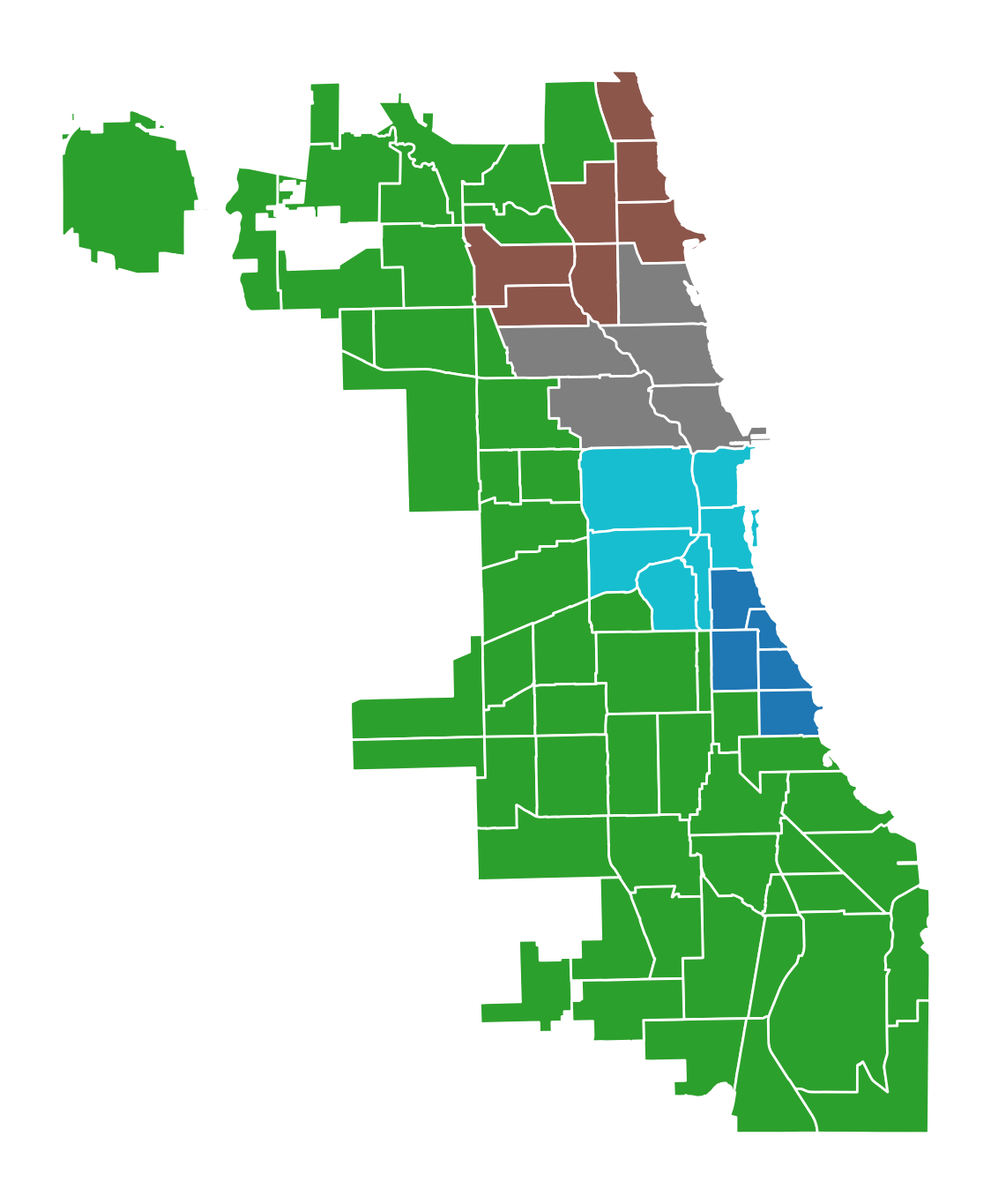The model solution results in five regions, two of which have five communities, one with six, one with seven, and one with fifty-four.

:

chicago["count"] = 1
chicago[["demo_regions", "count"]].groupby(by="demo_regions").count()

:

count
demo_regions
0 5
1 54
2 7
3 5
4 6

## 5. Exploring parameters¶

There are myriad parameter combinations that can be passed in for regionalizing with Skater. Here we will explore two combinations of parameters.

• Increasing n_clusters while holding all other parameters constant

• Increasing floor while holding all other parameters constant

• Clustering on multiple variables

### Increasing n_clusters¶

Let’s see how regionalization results differ when increasing n_clusters from 2 to 10 by increments of 2.

:

n_clusters_range = [2, 4, 6, 8, 10]
n_clusters_range

:

[2, 4, 6, 8, 10]


For each regionalization run we will hold the other parameters constant.

:

floor, trace, islands = 5, False, "increase"
spanning_forest_kwds = dict(
dissimilarity=skm.manhattan_distances,
affinity=None,
reduction=numpy.sum,
center=numpy.mean,
verbose=False
)


Solve.

:

for ncluster in n_clusters_range:
model = spopt.region.Skater(
chicago,
w,
attrs_name,
n_clusters=ncluster,
floor=floor,
trace=trace,
islands=islands,
spanning_forest_kwds=spanning_forest_kwds
)
model.solve()
chicago[f"clusters_{ncluster}"] = model.labels_


Plot results.

:

f, axarr = plt.subplots(1, len(n_clusters_range), figsize=(9, 7.5))
for ix, clust in enumerate(n_clusters_range):
label = f"clusters_{clust}"
chicago.plot(column=label, ax=axarr[ix], cmap="tab20")
axarr[ix].set_title(label)
axarr[ix].set_axis_off()
plt.tight_layout()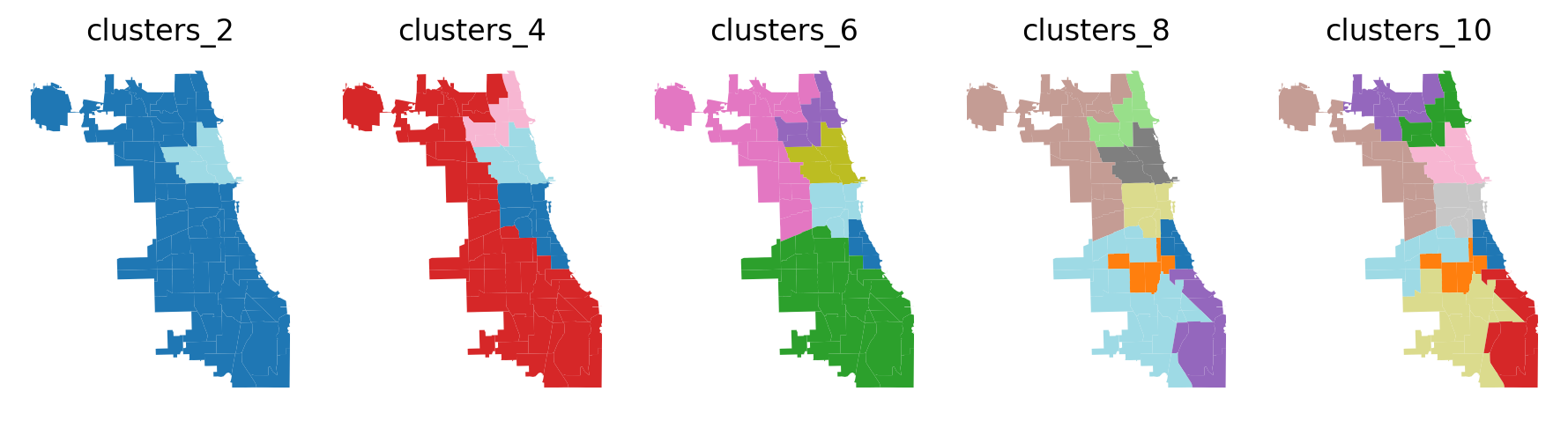Above we can see that regions become more dispersed and boundaries become more consistent as the number of desired clusters increases. Also, we can investigate the count of communities and the number of AirBnB spots per region, as shown below.

:

attr1, attr2 = "count", "num_spots"
df = pandas.DataFrame(
columns=pandas.MultiIndex.from_product(
[[f"clusters_{i}" for i in n_clusters_range], [attr1, attr2]]
),
index=range(10)
)
df.index.name = "region_id"
for i in n_clusters_range:
col = f"clusters_{i}"
df[col, attr1] = chicago[[col, attr1]].groupby(by=col).count()[attr1]
df[col, attr2] = chicago[[col, attr2]].groupby(by=col).sum()[attr2]
df.fillna(0).astype(int).astype(str).replace("0", "-")

:

clusters_2 clusters_4 clusters_6 clusters_8 clusters_10
count num_spots count num_spots count num_spots count num_spots count num_spots
region_id
0 72 2321 11 963 5 236 5 236 5 236
1 5 2705 54 429 35 143 5 38 5 38
2 - - 7 929 7 929 7 929 7 929
3 - - 5 2705 19 286 5 58 5 58
4 - - - - 5 2705 19 286 8 147
5 - - - - 6 727 5 2705 11 139
6 - - - - - - 6 727 5 2705
7 - - - - - - 25 47 6 727
8 - - - - - - - - 17 27
9 - - - - - - - - 8 20

### Increasing floor¶

Next we’ll increase floor from 3 to 15 by increments of 3.

:

floor_range = [3, 6, 9, 12, 15]
floor_range

:

[3, 6, 9, 12, 15]


For each regionalization run we will hold the other parameters constant.

:

n_clusters, trace, islands = 3, True, "increase"
spanning_forest_kwds = dict(
dissimilarity=None,
affinity=skm.euclidean_distances,
reduction=numpy.sum,
center=numpy.std,
verbose=False
)


Solve.

:

for floor in floor_range:
model = spopt.region.Skater(
chicago,
w,
attrs_name,
n_clusters=n_clusters,
floor=floor,
trace=trace,
islands=islands,
spanning_forest_kwds=spanning_forest_kwds
)
with warnings.catch_warnings():
# due to affinity
warnings.filterwarnings(
"ignore",
category=RuntimeWarning,
message="divide by zero encountered in log",
)
model.solve()
chicago[f"floor_{floor}"] = model.labels_


Plot results.

:

f, axarr = plt.subplots(1, len(floor_range), figsize=(9, 7.5))
for ix, floor in enumerate(floor_range):
label = f"floor_{floor}"
chicago.plot(column=label, ax=axarr[ix], cmap="tab20")
axarr[ix].set_title(label)
axarr[ix].set_axis_off()
plt.tight_layout()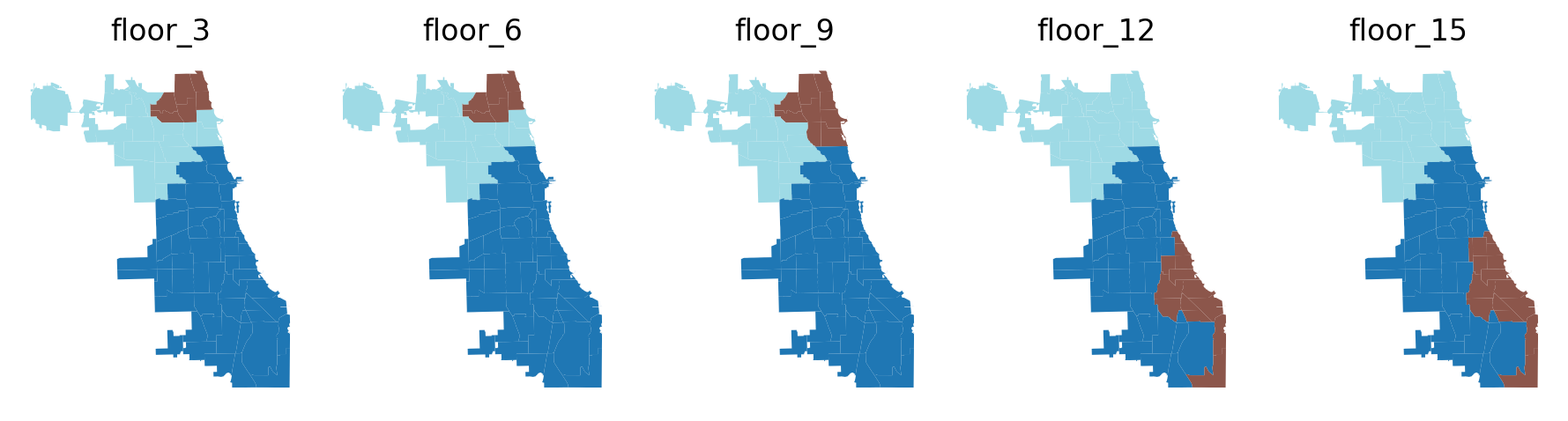Above we can see an interesting pattern. Boundaries are fixed when stipulating a minimum of 3 and 6 communities per region then the smallest region increases by 3 communities when stipulating floor==9. However, as the minimum number of communities is increased to 12 and 15 the location, shape, and rank of the 3 desired regions vary greatly. See the count of communities and the number of AirBnB spots per region below.

:

attr1, attr2 = "count", "num_spots"
df = pandas.DataFrame(
columns=pandas.MultiIndex.from_product(
[[f"floor_{i}" for i in floor_range], [attr1, attr2]]
),
index=range(3)
)
df.index.name = "region_id"
for i in floor_range:
col = f"floor_{i}"
df[col, attr1] = chicago[[col, attr1]].groupby(by=col).count()[attr1]
df[col, attr2] = chicago[[col, attr2]].groupby(by=col).sum()[attr2]
df

:

floor_3 floor_6 floor_9 floor_12 floor_15
count num_spots count num_spots count num_spots count num_spots count num_spots
region_id
0 52 2867 52 2867 52 2867 40 2639 33 2814
1 6 504 6 504 9 1440 12 228 25 2159
2 19 1655 19 1655 16 719 25 2159 19 53

### Clustering on more than one variable¶

First, let’s isolate some of the variables in the dataset that are characterisitc of social vulnerability.

:

attrs_name = [
"poverty",
"crowded",
"without_hs",
"unemployed",
"harship_in",
"num_crimes",
"num_theft"
]


Not all values are available in all communities for each variable, so we’ll set those to 0.

:

for i in attrs_name:
chicago[i] = chicago[i].fillna(0)


Now we’ll see how regionalization results differ when increasing n_clusters from 3 to 7 by increments of 1 while considering the 7 social vulnerability variables.

:

n_clusters_range = [3, 4, 5, 6, 7]
n_clusters_range

:

[3, 4, 5, 6, 7]


For each regionalization run we will hold the other parameters constant.

:

floor, trace, islands = 8, True, "increase"
spanning_forest_kwds = dict(
dissimilarity=skm.manhattan_distances,
affinity=None,
reduction=numpy.sum,
center=numpy.mean,
verbose=False
)


Solve.

:

for ncluster in n_clusters_range:
model = spopt.region.Skater(
chicago,
w,
attrs_name,
n_clusters=ncluster,
floor=floor,
trace=trace,
islands=islands,
spanning_forest_kwds=spanning_forest_kwds
)
model.solve()
chicago[f"clusters_{ncluster}"] = model.labels_


Plot results.

:

f, axarr = plt.subplots(1, len(n_clusters_range), figsize=(9, 7.5))
for ix, clust in enumerate(n_clusters_range):
label = f"clusters_{clust}"
chicago.plot(column=label, ax=axarr[ix], cmap="tab20")
axarr[ix].set_title(label)
axarr[ix].set_axis_off()